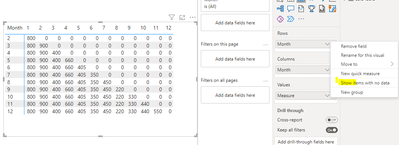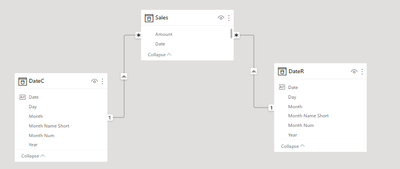cancel
Showing results for
Did you mean:Super User

## FILTER on second date table

Hi community!

I have a sales table and two date tables. The sales table is linked via Date column to both tables.

THen I have a matrix with these sales as a value. Then I have Months selected as columns in matrix from the first date table.

 Jan Feb Mar Apr May June ... ... ... ... 800 900 400 660 405 350 450 ... ... ...

Now I would like to add again Months as a row but from the second date table.

THis selected row determines the last date which should be considered for the matrix:

 Jan Feb Mar Apr May June ... ... ... ... Feb 800 0 0 0 0 0 0 0 0 0 Mar 800 900 0 0 0 0 0 0 0 0 Apr 800 900 400 0 0 0 0 0 0 0

How would you do that with DAX?

1 ACCEPTED SOLUTIONCommunity Support

Hi @joshua1990 ,

According to your description, here are my steps you can follow as a solution.

(1) My test data is the same as yours.

(2)My model relationship is as follows.(3) We can create two measures.

``````Measure =
var _a = IF(SELECTEDVALUE('CALENDAR Table 2'[Month])>SELECTEDVALUE('CALENDAR Table 1'[Month]) ,
CALCULATE(
SUM('sale Table'[sales]),
'CALENDAR Table 2'[Date] < MAX('CALENDAR Table 2'[Date])
),0)
return IF(SELECTEDVALUE('CALENDAR Table 2'[Month]) =1,BLANK(),_a)``````

(4) Then the result is as follows.Best Regards,

Neeko Tang

If this post  helps, then please consider Accept it as the solution  to help the other members find it more quickly.

4 REPLIES 4Super User

I've called the 2 date tables DateC (for the one to go on columns) and DateR (for the one to go on rows)When you create the matrix the filter coming from the column is fine as it is.  Your first table is getting you the correct figures per month.  The issue is the filter coming from the DateR table, so that's what we want to alter.

So, this measure alters that filter:

``````Sales Triangle =
CALCULATE(
SUM(Sales[Amount]),
DateR[Date] < MIN(DateR[Date])
)``````

and gives you this result (my base data is different, hence the different amounts)Community Support

Hi @joshua1990 ,

According to your description, here are my steps you can follow as a solution.

(1) My test data is the same as yours.

(2)My model relationship is as follows.(3) We can create two measures.

``````Measure =
var _a = IF(SELECTEDVALUE('CALENDAR Table 2'[Month])>SELECTEDVALUE('CALENDAR Table 1'[Month]) ,
CALCULATE(
SUM('sale Table'[sales]),
'CALENDAR Table 2'[Date] < MAX('CALENDAR Table 2'[Date])
),0)
return IF(SELECTEDVALUE('CALENDAR Table 2'[Month]) =1,BLANK(),_a)``````

(4) Then the result is as follows.Best Regards,

Neeko Tang

If this post  helps, then please consider Accept it as the solution  to help the other members find it more quickly.Super User

Any ideas? Do you need further information?Super User

No ideas?